# Convex Square Peg Problem

Mar. 15, 2022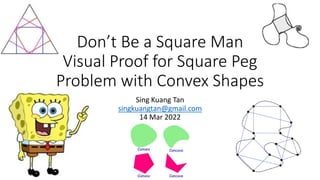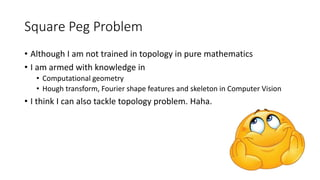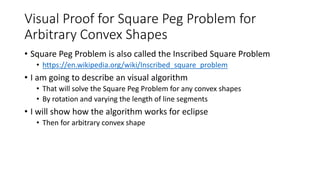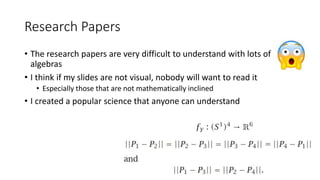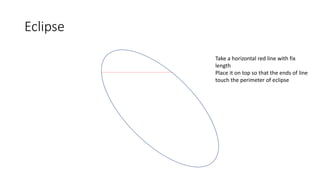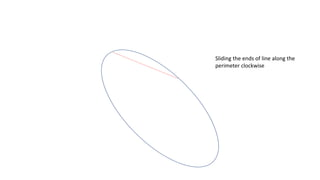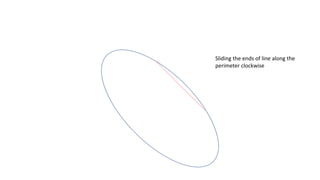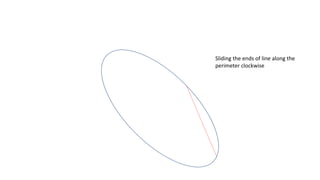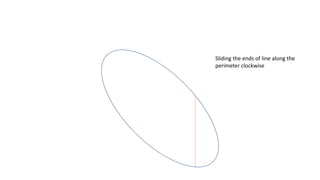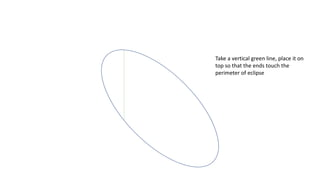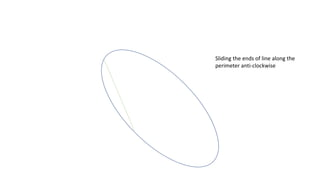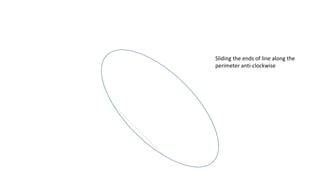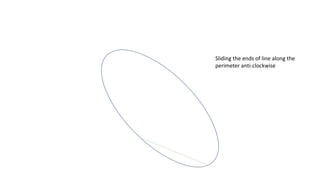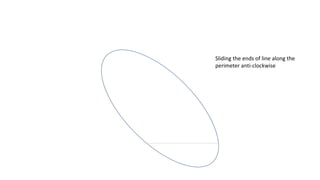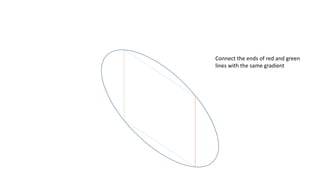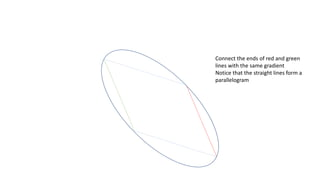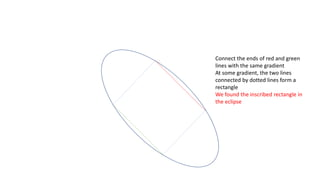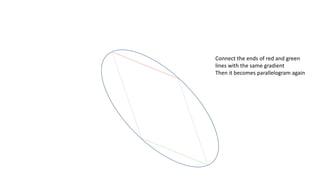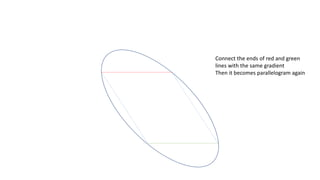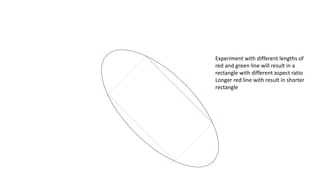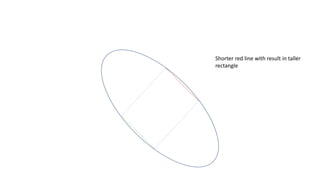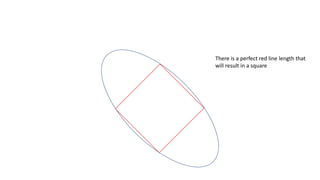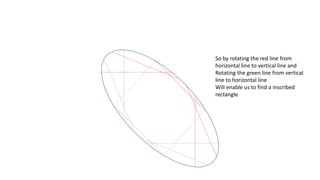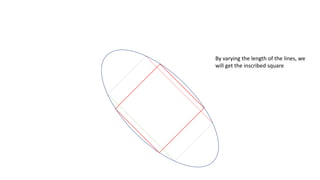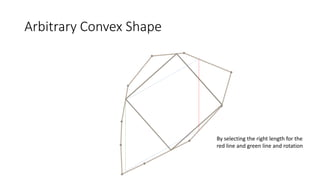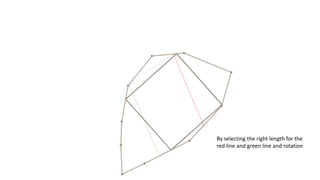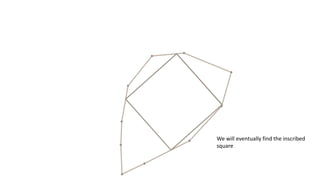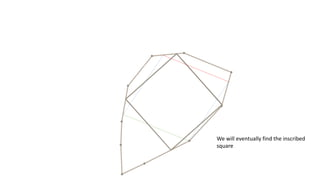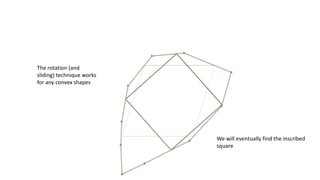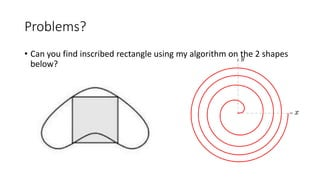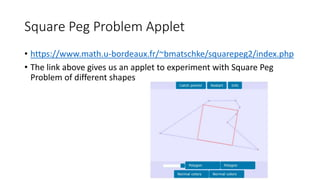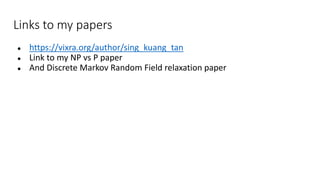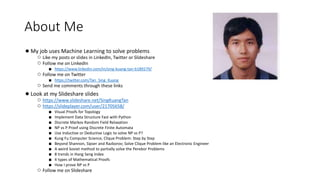1 of 34

### Convex Square Peg Problem

• 1. Don’t Be a Square Man Visual Proof for Square Peg Problem with Convex Shapes Sing Kuang Tan singkuangtan@gmail.com 14 Mar 2022
• 2. Square Peg Problem • Although I am not trained in topology in pure mathematics • I am armed with knowledge in • Computational geometry • Hough transform, Fourier shape features and skeleton in Computer Vision • I think I can also tackle topology problem. Haha.
• 3. Visual Proof for Square Peg Problem for Arbitrary Convex Shapes • Square Peg Problem is also called the Inscribed Square Problem • https://en.wikipedia.org/wiki/Inscribed_square_problem • I am going to describe an visual algorithm • That will solve the Square Peg Problem for any convex shapes • By rotation and varying the length of line segments • I will show how the algorithm works for eclipse • Then for arbitrary convex shape
• 4. Research Papers • The research papers are very difficult to understand with lots of algebras • I think if my slides are not visual, nobody will want to read it • Especially those that are not mathematically inclined • I created a popular science that anyone can understand
• 5. Eclipse Take a horizontal red line with fix length Place it on top so that the ends of line touch the perimeter of eclipse
• 6. Sliding the ends of line along the perimeter clockwise
• 7. Sliding the ends of line along the perimeter clockwise
• 8. Sliding the ends of line along the perimeter clockwise
• 9. Sliding the ends of line along the perimeter clockwise
• 10. Take a vertical green line, place it on top so that the ends touch the perimeter of eclipse
• 11. Sliding the ends of line along the perimeter anti-clockwise
• 12. Sliding the ends of line along the perimeter anti-clockwise
• 13. Sliding the ends of line along the perimeter anti-clockwise
• 14. Sliding the ends of line along the perimeter anti-clockwise
• 15. Connect the ends of red and green lines with the same gradient
• 16. Connect the ends of red and green lines with the same gradient Notice that the straight lines form a parallelogram
• 17. Connect the ends of red and green lines with the same gradient At some gradient, the two lines connected by dotted lines form a rectangle We found the inscribed rectangle in the eclipse
• 18. Connect the ends of red and green lines with the same gradient Then it becomes parallelogram again
• 19. Connect the ends of red and green lines with the same gradient Then it becomes parallelogram again
• 20. Experiment with different lengths of red and green line will result in a rectangle with different aspect ratio Longer red line with result in shorter rectangle
• 21. Shorter red line with result in taller rectangle
• 22. There is a perfect red line length that will result in a square
• 23. So by rotating the red line from horizontal line to vertical line and Rotating the green line from vertical line to horizontal line Will enable us to find a inscribed rectangle
• 24. By varying the length of the lines, we will get the inscribed square
• 25. Arbitrary Convex Shape By selecting the right length for the red line and green line and rotation
• 26. By selecting the right length for the red line and green line and rotation
• 27. We will eventually find the inscribed square
• 28. We will eventually find the inscribed square
• 29. We will eventually find the inscribed square The rotation (and sliding) technique works for any convex shapes
• 30. Problems? • Can you find inscribed rectangle using my algorithm on the 2 shapes below?
• 31. Square Peg Problem Applet • https://www.math.u-bordeaux.fr/~bmatschke/squarepeg2/index.php • The link above gives us an applet to experiment with Square Peg Problem of different shapes
• 32. Links to my papers ● https://vixra.org/author/sing_kuang_tan ● Link to my NP vs P paper ● And Discrete Markov Random Field relaxation paper